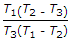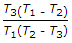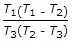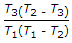# Mechanical Engineering - Heat Transfer, Refrigeration and Air Conditioning

Exercise : Heat Transfer, Refrigeration and Air Conditioning - Section 1
1.
The wet bulb temperature during sensible heating of air
remains constant
increases
decreases
Explanation:
No answer description is available. Let's discuss.

2.
The C.O.P. of an absorption type refrigerator is given by (where T1 = Temperature at which the working substance receives heat, T2 = Temperature of cooling water, and T3 = Evaporator temperature)Explanation:
No answer description is available. Let's discuss.

3.
Which of the following statement is wrong?
The heat transfer in liquid and gases takes place according to convection.
The amount of heat flow through a body is dependent upon the material of the body.
The thermal conductivity of solid metals increases with rise in temperature,
Logarithmic mean temperature difference is not equal to the arithmetic mean temperature difference.
Explanation:
No answer description is available. Let's discuss.

4.
In free convection heat transfer transition from laminar to turbulent flow is governed by the critical value of the
Reynold's number
Grashoff's number
Reynold's number, Grashoff's number
Prandtl number, Grashoff's number